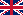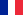This site uses Google Analytics to make statistics on the pages consulted and to detect possible dysfunctions.
However, this site functions perfectly without cookies. You can therefore deactivate them.

Euroballistics - The expertise
The recoil of weapons
Development of high velocity small caliber
 HomeEnglish /Français Contact
 THE RECOIL OF WEAPONS AND DEVELOPMENT OF HIGH VELOCITY SMALL CALIBER
 Jean-Jacques DÖRRZAPF

The recoil of weapons is an important phenomenon that has been studied extensively because it subjects them to significant mechanical stress. The shooter is also affected by this phenomenon as well as the accuracy of the shot in the case of high rate shooting, especially in bursts.

The modeling of the efficiency of a firearm has been the subject of numerous researches without any compromise or equation, sometimes esoteric, having really accounted for the phenomenon (read on this subject the article - currently in French - ) of Serge LOPEZ.

For our part, taking into account the experiments that we have conducted as well as those carried out by various ballistics laboratories, we consider that the lesion potential of a weapon is directly related to the kinetic energy of the projectile. This is a tangible, measurable and verifiable fact, both experimentally and through feedback from the field.

We present below a method to define the recoil of weapons with a good approximation and to show a possibility to minimize this phenomenon for a kinetic energy imposed by the target characteristics.

I – RECOIL REACTION

Let consider the following parameters :

•  V0 : initial velocity of the projectile ;
•  mp : mass of the projectile ;
•  KE : kinetic energy of the projectile ;
•  mpd : mass of powder ;
•  v0 : initial recoil velocity of the recoiling mass ;
•  M : recoiling mass.

At the moment when the projectile will leave the tube, the law of conservation of momentum allows to write :(1)

Note on recoil parameters :

1) About gases velocity : The velocity of the gases is considered with a good approximation to be, on average, half that of the projectile at the moment when the latter leaves the tube. However, on weapons with a chamber (long guns) this velocity is slightly less than half that of the projectile. In fact, since the chamber is larger in diameter than the tube, the percentage of gas still in the chamber is greater than that moving behind the projectile.

2) On the phenomena leading to the recoil : In order to model the phenomenon as closely as possible, one can add, to the first member of equation (1), a corrective term G representing the impulse resulting from the escape of gases through the weapon's muzzle, thus creating a small additional blowing effect and inducing an increase in the weapon's recoil. However, formula (1) as it stands gives an good approximation.

II – FORMULATION OF THE RECOIL OF THE WEAPON AS A FUNCTION OF THE KINETIC ENERGY OF THE       PROJECTILE

The formulation of the weapon's recoil as a function of the kinetic energy with which we wish to endow the projectile can allow us, by playing on the mass/velocity pair of the projectile, to minimize the importance of the recoil for a fixed kinetic energy.

1. The equivalence between kinetic energy and mechanical work (product of a force and a distance x) :leads us to consider that the wounding effects are proportional to the kinetic energy of the projectile ;

2. As a first approximation, the kinetic energy of the projectile is proportional to the mass of powder :(2)

The initial kinetic energy of the projectile is given by the relation :(3)

By introducing the kinetic energy of the projectile KE in the expression of the momentum of the weapon Mv0 ,
we obtain :soit :(4)

having noticed that :etThe expression (4) gives us the value of the momentum of the weapon as a function of the kinetic energy of the projectile.

For a given kinetic energy, Mv0 will vary according to the initial velocity of the projectile. We must therefore look for a value of V0 for which we will have a minimum for the expression (4) whose graphical representation appears below.For a given kinetic energy, the momentum related to the recoil mass will be minimal when the derivative of expression (4) is zero. Since we are looking for a value of V0, this value must be treated as a variable.

That is, :orhenceIII – NUMERIC APPLICATIONS

III. I - La munition de 5,56 x 45 mm (.223 Remington)

The data :

- mp = 3,56 g ;

- mpd = 1,63 g ;

- V0 = 975 m/s ;

We obtain KE = 1692 J, hence V0 = 2038 m/s, mp = 0,8 g et Mv0 = 3,3 Ns for a minimum recoil.

The momentum generated by the 5.56 x 45 mm ammunition is 4.27 Ns, which is 31% higher than that generated by the "ideal" ammunition.

 Momentum of the 5.56x45 mm ammunition as a function of V0 for a given KETechnical difficulties :

− Existence of a powder capable of propelling a projectile at the calculated velocity ;

− Tube wear, projectile jacket/barrel grooves interaction, etc...

III. II - 9 mm Parabellum ammunition

The data :

− mp = 8 g ;

−  m
pd = 0,4 g ;

−  V
0 = 350 m/s ;

We obtain KE = 490 J, hence V0 = 2214 m/s, mp = 0,2 g et Mv0 = 0,89 Ns for a minimum recoil.

The momentum of the 9 mm Parabellum ammunition is 2.87 Ns, 220% higher than that generated by the "ideal" ammunition.

 Momentum of the 9 mm Parabellum ammunition as a function of V0 for a given KETechnical difficulties :

The same as described for the previous ammunition.

However, the creation, in the 1980s, of THV (Très Haute Vitesse - Very High Velocity) hand guns ammunition by the Société Française de Muniitons (mp = 2,9 g, V0 ≈ 700 m/s, mpd = 0,44 g, KE = 711 J ) is justified by the calculation.

IV – CONCLUSION

The calculation shows that for a given kinetic energy, the increase in velocity and the correlative decrease in mass tend to minimize the recoil of a weapon. The limits are imposed by technical constraints. This finding is interesting from an ergonomic point of view but also for the stability of individual weapons firing at a high rate.

If we consider (as demonstrated by the laws of mechanics and experiments) that the volume of lesions is proportional to the kinetic energy of the projectile, it appears that small projectiles with a high velocity can generate significant lesions that need not be attributed, due to a lack of knowledge of the underlying interactions, to a few phenomena that are often myths, such as that of the shock wave, which cannot exist given that projectiles in biological tissues propagate at subsonic velocities in relation to these media.

 Home Contact
 @ : contact@euroballistics Copyright : EuroBallistics/JJ Dorrzapf - 2006-2023